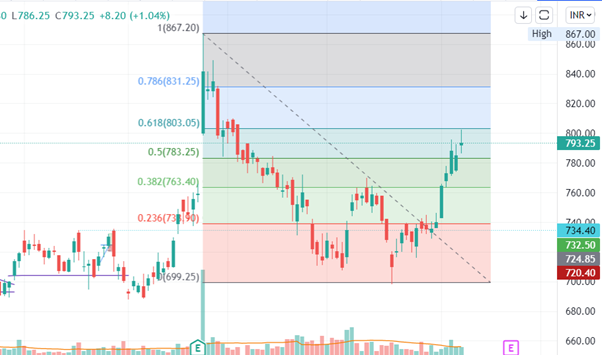Finschool By 5paisa

• #
• A
• B
• C
• D
• E
• F
• G
• H
• I
• J
• K
• L
• M
• N
• O
• P
• Q
• R
• S
• T
• U
• V
• W
• X
• Y
• Z

# Fibonacci Retracement# Fibonacci Retracement

A Fibonacci retracement is a technical indicator used to identify support and resistance levels in a time series of prices or index levels. Unlike many technical indicators, Fibonacci retracements cannot be used directly to generate buy and sell signals. Instead, they are used as guides in conjunction with other indicators to make trading decisions.

##### The Fibonacci sequence

Why are Fibonacci retracements named as such? The indicator derives its name from the well-known mathematical sequence called the Fibonacci sequence. It is a simple sequence that can be generated from two basic rules:

The first two elements of the sequence are 0 and 1, respectively.

Further terms are generated as the sum of the preceding two elements.

The following formula summarizes the sequence-Using the formula, we get the following sequence:

{0,1,1,2,3,5,8,13,21,34,55,89,….}

##### How to use the Fibonacci retracement levels?

Think of a situation where you wanted to buy a particular stock, but you have not been able to do so because of a sharp run-up in the stock. The most prudent action to take would be to wait for a retracement in the stock in such a situation. Fibonacci retracement levels such as 61.8%, 38.2%, and 23.6% act as a potential level up to which a stock can correct.

By plotting the Fibonacci retracement levels, the trader can identify these retracement levels, and therefore position himself for an opportunity to enter the trade. However please note like any indicator, use the Fibonacci retracement as a confirmation tool.

I would buy a stock only after it has passed the other checklist items. In other words, my conviction to buy would be higher if the stock has:

Formed a recognizable candlestick pattern

The stop loss coincides with the S&R level.

Volumes are above average.

Along with the above points, if the stop loss also coincides with the Fibonacci level, I know the trade setup is well aligned to all the variables, and hence I would go in for a strong buy. The word ‘strong’ usage indicates the level of conviction in the trade set up. The more confirming factors we use to study the trend and reversal, more robust is the signal. The same logic can also be applied for the short trade.

##### Fibonacci Retracement construction

Step 1- Identify immediate peak and trough. In this case, the trough is at 150, and the peak is at 240. The 90 point moves make it 100%.Step 2- Select the Fibonacci retracement tool from the chart toolsStep 3- Use the Fibonacci retracement tool to connect the trough and the peak.After selecting the Fibonacci retracement tool from the charts tool, the trader has to click on trough first, and without un-clicking, he has to drag the line till the peak. While doing this, simultaneously, the Fibonacci retracements levels start getting plotted on the chart. However, the software completes the retracement identification process only after selecting both the trough and the peak. This is how the chart looks after selecting both points.

View All